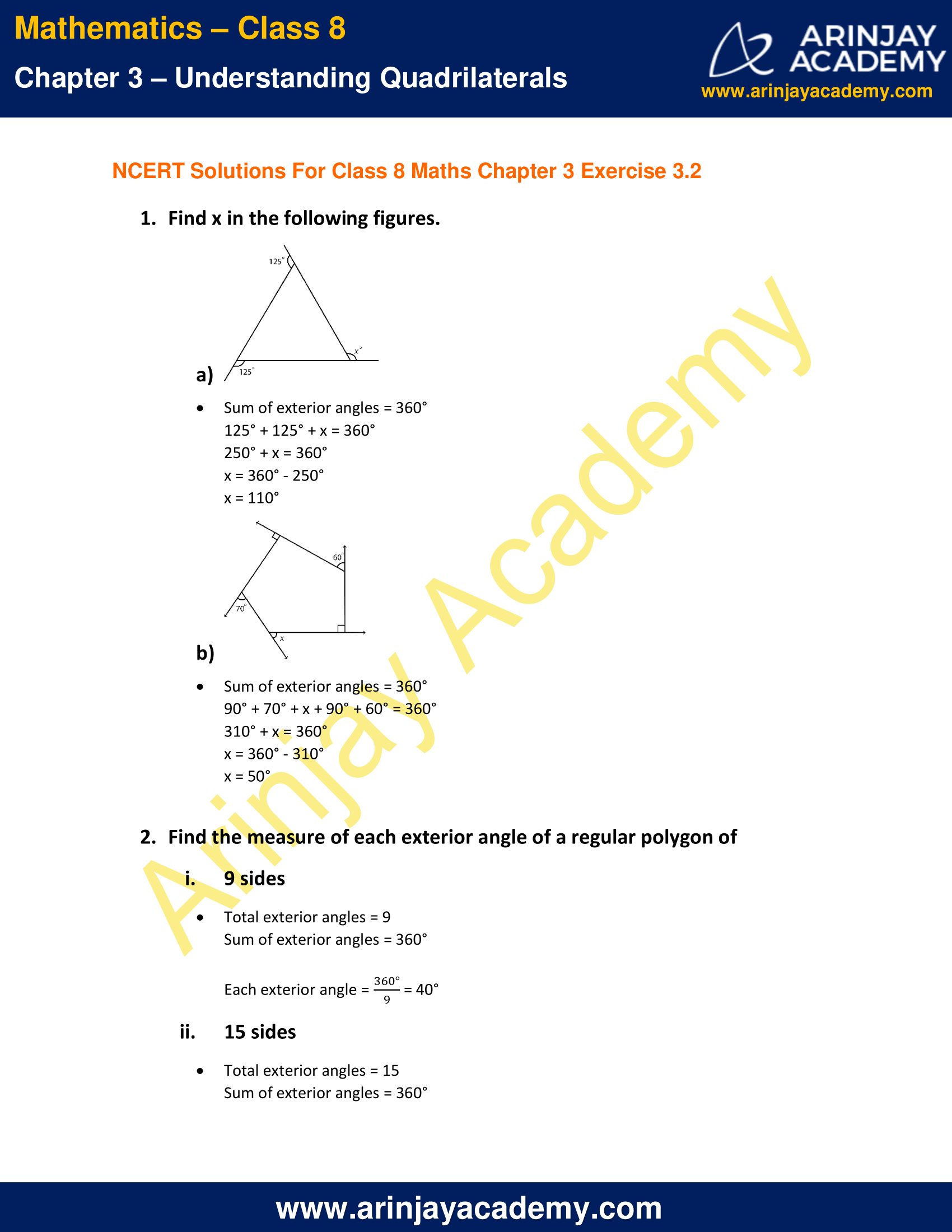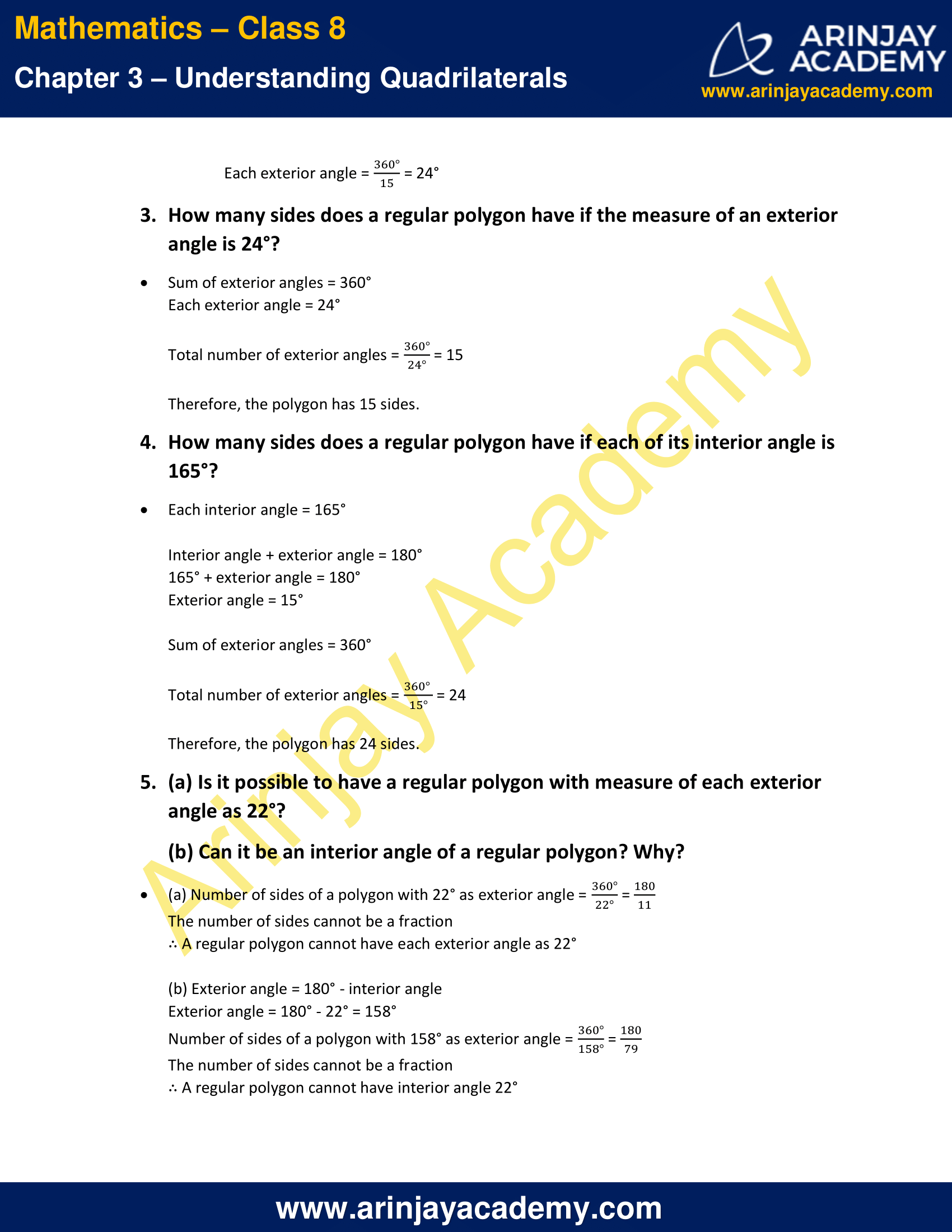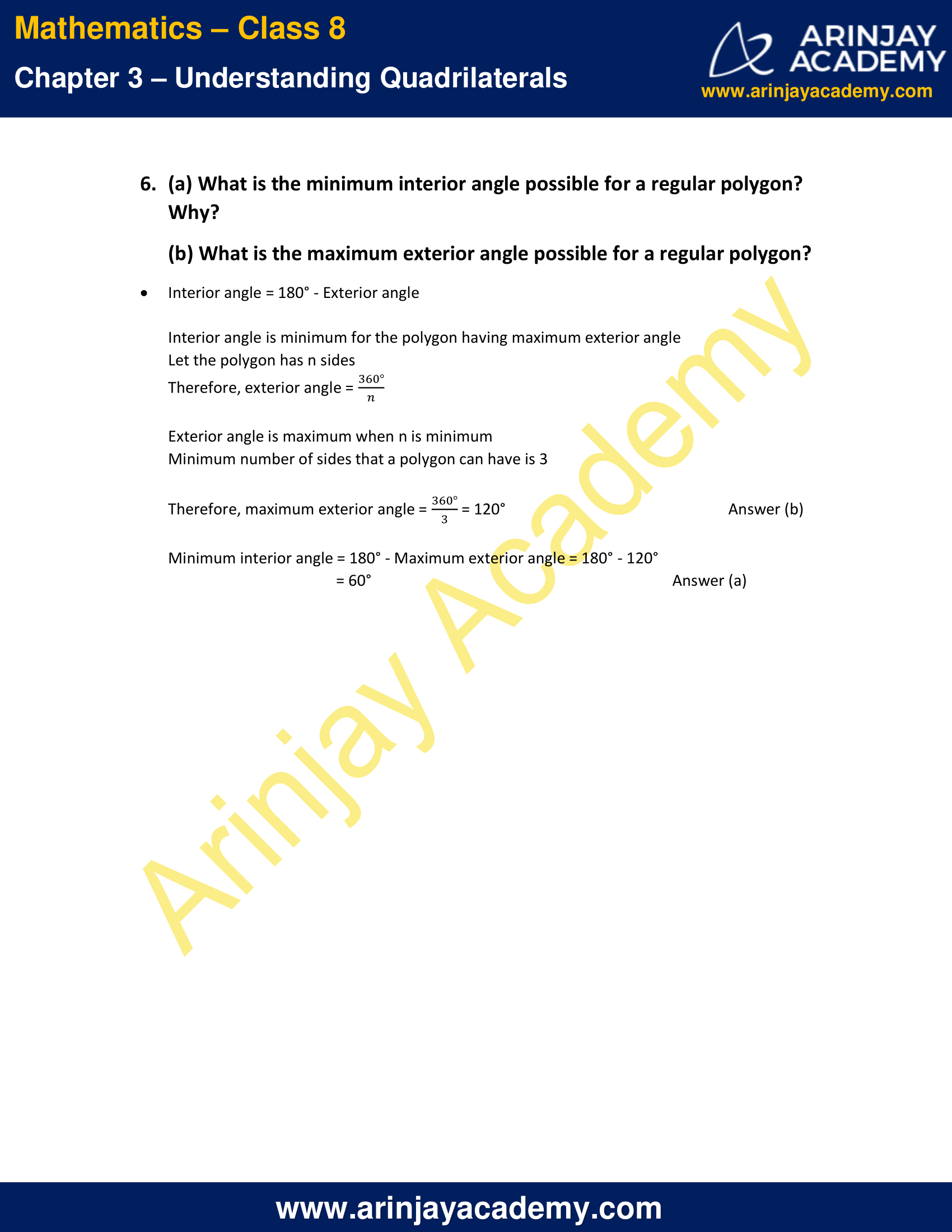# NCERT Solutions for Class 8 Maths Chapter 3 Exercise 3.2

NCERT Solutions for Class 8 Maths Chapter 3 Exercise 3.2 – Understanding Quadrilaterals, has been designed by the NCERT to test the knowledge of the student on the topic – Sum of the Measures of the Exterior Angles of a Polygon

### NCERT Solutions for Class 8 Maths Chapter 3 Exercise 3.2NCERT Solutions for Class 8 Maths Chapter 3 Exercise 3.2

1. Find x in the following figures.

a)Solution:

Sum of exterior angles = 360°
125° + 125° + x = 360°
250° + x = 360°
x = 360° – 250°
x = 110°

b)Solution:

Sum of exterior angles = 360°
90° + 70° + x + 90° + 60° = 360°
310° + x = 360°
x = 360° – 310°
x = 50°

Find the measure of each exterior angle of a regular polygon of

i) 9 sides

Solution:

Total exterior angles = 9
Sum of exterior angles = 360°
Each exterior angle = (360°/9) = 40°

ii) 15 sides

Solution:

Total exterior angles = 15
Sum of exterior angles = 360°
Each exterior angle = (360°/15) = 24°

3. How many sides does a regular polygon have if the measure of an exterior angle is 24°?

Solution:

Sum of exterior angles = 360°
Each exterior angle = 24°
Total number of exterior angles = (360°/24°) = 15
Therefore, the polygon has 15 sides.

7. How many sides does a regular polygon have if each of its interior angle is 165°?

Solution:

Each interior angle = 165°
Interior angle + exterior angle = 180°
165° + exterior angle = 180°
Exterior angle = 15°
Sum of exterior angles = 360°
Total number of exterior angles = (360°/15°) = 24
Therefore, the polygon has 24 sides.

5. (a) Is it possible to have a regular polygon with measure of each exterior angle as 22°?
(b) Can it be an interior angle of a regular polygon? Why?

Solution:

(a) Number of sides of a polygon with 22° as exterior angle = (360°/22°) = (180/11)
The number of sides cannot be a fraction
∴ A regular polygon cannot have each exterior angle as 22°

(b) Exterior angle = 180° – interior angle
Exterior angle = 180° – 22° = 158°
Number of sides of a polygon with 158° as exterior angle = (360°/158°) = (180/79)
The number of sides cannot be a fraction
∴ A regular polygon cannot have interior angle 22°

6. (a) What is the minimum interior angle possible for a regular polygon? Why?
(b) What is the maximum exterior angle possible for a regular polygon?

Solution:

Interior angle = 180° – Exterior angle
Interior angle is minimum for the polygon having maximum exterior angle
Let the polygon has n sides
Therefore, exterior angle = (360°/n)
Exterior angle is maximum when n is minimum
Minimum number of sides that a polygon can have is 3
Therefore maximum exterior angle = (360°/3) = 120°   Answer (b)
Minimum interior angle
= 180° – Maximum exterior angle
= 180° – 120°